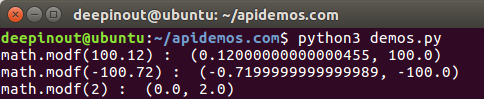# Python Math log() 函数

## Python Math log() 函数

log() 方法返回x的自然对数，x > 0。

## Python Math log() 语法

``````import math

math.log( x )``````

• x - 数值表达式。

## Python Math log() 示例1

``````#!/usr/bin/python3

import math

print ("math.log(100.12) : ", math.log(100.12))
print ("math.log(100.72) : ", math.log(100.72))
print ("math.log(math.pi) : ", math.log(math.pi))``````• 微信订阅
• 回顶